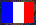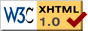Version française

# Hexcolumns, version 1.01 (1995-02-23)

Note: this is the help text extracted from the rule Hexcolumns. You need to read a part of TETRHEX help to understand everything.

## Presentation

Hexcolumns is a hexagonal columns, that is played on a hexagonal board whose edge has 6, 7, 8 or 9 hexagons. The pieces are composed of 3 vertically arranged little hexagons; each hexagon has one of the 6 colors (with equal probabilities). The aim is to align 3 (or more) hexagons of the same color, in one of the 3 directions. But some hexagons have a special behavior, as the pattern indicates it. The pieces appear at the top (in the center) and fall at a speed that depends only upon the level; you lose when the next piece can't appear.

There are 5 kinds of patterns: the +, the -, the *, the bomb and the square. There is a 1/10 chance that a hexagon has a pattern. If it is the case, there is a 2/3 chance that this pattern is a square, and a 1/12 chance for each of the other 4 patterns.

• The +: another hexagon must be aligned (i.e. 4 hexagons if there is 1 +, 5 hexagons if there are 2 +...), or 4 (or more) + of any colors must be aligned. All the + are destroyed when the score reaches a multiple of 2500n - 12500, where n is the number of the cells in an edge of the hexagonal board (10000 if n = 9, 7500 if n = 8, 5000 if n = 7, 2500 if n = 6).
• The -: this hexagon is in any alignment, except in alignments of its color, as if it were a hexagon with no pattern having each of the other 5 colors.
• The *: this hexagon is destroyed, and also destroys its 6 possible neighbors.
• The bomb: this hexagon can't be in any alignment; it explodes after a while: each time a piece arrives, it has one chance in 30 of exploding. If it explodes, each neighbor has one chance over 2 to explode.
• The square: this hexagon can't be in any alignment, but when there are 4 squares (or more) on the board, they are destroyed.

The destructions don't happen at the same time; thus their order is very important. Here is it: * explosion, bomb explosion, square destruction, + destruction (when the score reaches a given number), alignments. Then the score is updated (not before).

The pieces can be moved

• in V (keys: left and right arrows), i.e. to the left or to the right at the same height; the height is by definition the number of the hexagons that are below;
• downwards to the left (8 o'clock) or to the right (4 o'clock); keys: Insert and Clr-Home;
• downwards (A on a QWERTY keyboard, Q on an AZERTY keyboard); in this case, the piece won't fall for the saved time. Note: this move isn't very useful!

You also can do a downwards circular permutation of the 3 hexagons. Note: an upwards circular permutation is obtained with 2 downwards circular permutations. You can drop a piece with the space bar to save time.

The Help key allows to switch on and off the radar (the next piece is displayed). When the radar is switched on, 200 points are subtracted from the score (the score is set to 0 if it was less than 200). When the radar is on, the destructions yield fewer points (see Section calculating the score).

The Undo key allows to pause. But the time goes on passing (see beneath). The Return key allows to continue.

Each level lasts 60 seconds. When the time (displayed at the bottom) reaches 0, the level is increased at the next piece (except for level 25). You can increase the level with the + key of the numeric pad; then the remaining time increases by 60 seconds. Thus, instead of spending one minute for each level starting at level 0, for instance you can select level 10, spend 11 minutes at level 10, then one minute at each following level.

To abort (whether the game is paused or not), type Ctrl-Esc.

## Calculating the Score

Each piece yields 5 points. The hexagon destruction yields a number of points of the form N × (k+L), where L is the level, k = 10 if the radar is off, k = 7 if the radar is on, and N is calculated in the following way:

• For the destructions different from alignments: each destroyed hexagon yields 1 point for N; in the case of bombs (and only in this case), a hexagon may be counted several times.
• For the alignments: after a destruction, other alignments may appear; thus the destruction may occur in several steps. A destroyed hexagon yields 2s, where s is the number of the step, i.e. 2 for the first step, 4 for the second one, 8 for the third one, and so on.Last updated:
• XML source: 2003-06-18, 23:39:56 (20)
• XSL stylesheet: 2003-08-06, 22:11:54 (488)
webmaster@vinc17.org